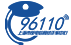• 上证- - -(涨:-平:- 跌:-)深证- - -(涨:-平:- 跌:-)

-

- -

• 今开:-
• 最高:-
• 买入价:-
• 成交量:-
• 仓差:-
• 昨结算:-
• 涨停:-
• 外盘:-
• 昨收:-
• 最低:-
• 卖出价:-
• 持仓量:-
• 日增:-
• 成交额:-
• 跌停:-
• 内盘:-
• 10:23• 主要指标
• 均线
• EXPMA
• BOLL
• SAR
• BBI
• RSI
• KDJ
• MACD
• W&R
• DMI
• BIAS
• OBV
• CCI
• ROC
• CR
• BOLL
• RSI
• KDJ
• MACD
• W&R
• DMI
• BIAS
• OBV
• CCI
• ROC
• CR
• BOLL
 交易品种 - 交易代码 - 交易单位 - 报价单位 - 上市交易所 - 最小变动价格 - 跌涨停板幅度 - 合约交割月份 - 交易时间 - 最后交易日 - 最后交割日 - 交割品级 - 最初交易保证金 - 交割方式 -

• ### 盈亏分析

• 成交量龙虎榜
名次 会员简称 成交量 增减
暂无数据
• 多头持仓龙虎榜
名次 会员简称 成交量 增减
暂无数据
• 空头持仓龙虎榜
名次 会员简称 成交量 增减
暂无数据

### 纯碱101吧